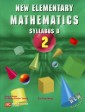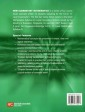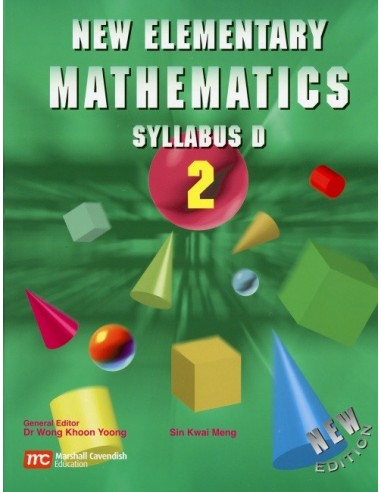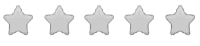See inside this book

# Singapore Math Grade 8 Textbook (level 2)

\$32.00
No tax
Math
8
P-MA08-51Leave a Review

#### Description

New Elementary Mathematics (Syllabus D) is a series in which emphasis is placed on the development of better understanding of mathematical concepts and their applications, as well as on proficiency in problem solving, mathematical reasoning and higher order thinking. It integrates the basics of higher math, pre-algebra, algebra, geometry, and some advanced math topics.

To facilitate this, the series has included the following:

• Investigative work
• Communication skills in mathematics and
• Appropriate computation and estimation skills
• Mental calculation
• Problem-solving heuristics

It is hoped that this series will help students gain confidence in and have better insights into the subject, and above all, that students will look upon Mathematics as something exciting, rewarding and useful.

New Elementary Math Textbook 2 (8th grade) teaches Indices, Algebraic Manipulations, Literal and Quadratic Equations, Word Problems, Graphs, Simultaneous Linear Equations, Inequalities, Congruent and Similar Triangles, Mensuration, Pythagoras' Theorem and Trigonometry, Motion Geometry, and Statistics. Copyright 2003. Dimensions 7.5 x 10 Inches. Softcover. 444 pp.